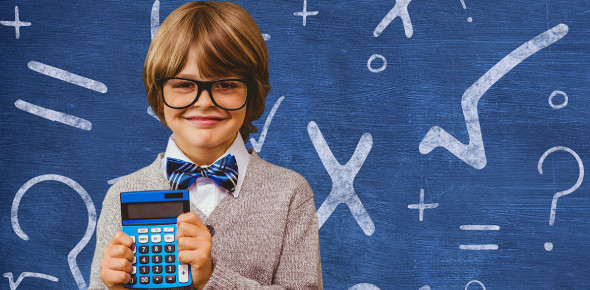# Maths Quiz For Class 3

10 Questions | Total Attempts: 103055SettingsDo you love to solve math problems? Take this quiz to see if you're a true maths champion or not. We've designed here a simple yet interesting quiz that consists of 3rd-grade maths questions. So, are you ready to test your brain cells? Let's get started then. All the best!

• 1.
Ram had 342 coins in his collection. How would you write 342?
• A.

Three four two

• B.

Three forty two

• C.

Three hundred forty two

• D.

Three hundred four two

• 2.
A shop keeper puts an even number of chocolates in a jar. How many chocolates could he have put in the pot?
• A.

999

• B.

900

• C.

901

• D.

905

• 3.
Which number would make the number sentence correct? * > 789
• A.

798

• B.

700

• C.

704

• D.

740

• 4.
Hari writes the number sentence given below: 90 + 41 = * + 90.Which number would go where the * is?
• A.

0

• B.

1

• C.

51

• D.

41

• 5.
Mrs. Geetha writes the following number sentence on the board:  600 X  * = 0. Which options would go where the * is ?
• A.

0

• B.

1

• C.

0/600

• D.

1/600

• 6.
Which of these number sentences is correct?
• A.

456 > 465

• B.

476 > 467

• C.

403 > 430

• D.

457 > 475

• 7.
Which number comes next in the series? 4231, 4331, 4431, ?
• A.

4432

• B.

4430

• C.

4531

• D.

5431

• 8.
Which of the following is in ascending order?
• A.

5234, 5243, 5324, 5342

• B.

5234, 5324, 5342, 5243

• C.

5342, 5324, 5243, 5234

• D.

5324, 5342, 5234, 5243

• 9.
Ram is reading a storybook containing 260 pages. He has completed reading 180 pages. How more pages should he read to finish the book?
• A.

60

• B.

80

• C.

40

• D.

120

• 10.
Rita had \$150. She wanted to buy a doll that costs \$230. How much more money does she need to buy the toy?
• A.

80

• B.

90

• C.

100

• D.

120

Related TopicsBack to top